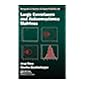Normal view MARC view ISBD view

Patterned random matrices

Material type:BookPublisher: Florida CRC Press 2018Description: xxi, 267p. With index.ISBN: 9781138591462.DDC classification: 512.9434 Summary: Large dimensional random matrices (LDRM) with specific patterns arise in econometrics, computer science, mathematics, physics, and statistics. This book provides an easy initiation to LDRM. Through a unified approach, we investigate the existence and properties of the limiting spectral distribution (LSD) of different patterned random matrices as the dimension grows. The main ingredients are the method of moments and normal approximation with rudimentary combinatorics for support. Some elementary results from matrix theory are also used. By stretching the moment arguments, we also have a brush with the intriguing but difficult concepts of joint convergence of sequences of random matrices and its ramifications. This book covers the Wigner matrix, the sample covariance matrix, the Toeplitz matrix, the Hankel matrix, the sample autocovariance matrix and the k-Circulant matrices. Quick and simple proofs of their LSDs are provided and it is shown how the semi-circle law and the Marchenko-Pastur law arise as the LSDs of the first two matrices. Extending the basic approach, we also establish interesting limits for some triangular matrices, band matrices, balanced matrices, and the sample autocovariance matrix. We also study the joint convergence of several patterned matrices, and show that independent Wigner matrices converge jointly and are asymptotically free of other patterned matrices. https://www.crcpress.com/Patterned-Random-Matrices/Bose/p/book/9781138591462
Tags from this library: No tags from this library for this title.
average rating: 0.0 (0 votes)
Item type Current location Item location Collection Call number Status Date due Barcode
Books Vikram Sarabhai Library
General Stacks
Slot 1368 (0 Floor, East Wing) Non-fiction 512.9434 B6P2 (Browse shelf) Available 199347
Browsing Vikram Sarabhai Library Shelves , Shelving location: General Stacks , Collection code: Non-fiction Close shelf browser
 No cover image available512.896 G3M2 Matrix algebra for engineers 512.897 S8I61 Introduction to linear algebra 512.9434 B6L2 Large covariance and autocovariance matrices 512.9434 B6P2 Patterned random matrices 512.9434 B6R2 Random circulant matrices 512.9434 M2M2 Matrix differential calculus with applications in statistics and econometrics 512.9434 T2T6 Topics in random matrix theory

1 A unified framework
2 Common symmetric patterned matrices
3 Patterned XX matrices
4 k-Circulant matrices
5 Wigner-type matrices
6 Balanced Toeplitz and Hankel matrices
7 Patterned band matrices
8 Triangular matrices
9 Joint convergence of i.i.d. patterned matrices
10 Joint convergence of independent patterned matrices
11 Autocovariance matrix

Large dimensional random matrices (LDRM) with specific patterns arise in econometrics, computer science, mathematics, physics, and statistics. This book provides an easy initiation to LDRM. Through a unified approach, we investigate the existence and properties of the limiting spectral distribution (LSD) of different patterned random matrices as the dimension grows. The main ingredients are the method of moments and normal approximation with rudimentary combinatorics for support. Some elementary results from matrix theory are also used. By stretching the moment arguments, we also have a brush with the intriguing but difficult concepts of joint convergence of sequences of random matrices and its ramifications. This book covers the Wigner matrix, the sample covariance matrix, the Toeplitz matrix, the Hankel matrix, the sample autocovariance matrix and the k-Circulant matrices. Quick and simple proofs of their LSDs are provided and it is shown how the semi-circle law and the Marchenko-Pastur law arise as the LSDs of the first two matrices. Extending the basic approach, we also establish interesting limits for some triangular matrices, band matrices, balanced matrices, and the sample autocovariance matrix. We also study the joint convergence of several patterned matrices, and show that independent Wigner matrices converge jointly and are asymptotically free of other patterned matrices.

https://www.crcpress.com/Patterned-Random-Matrices/Bose/p/book/9781138591462

There are no comments for this item.